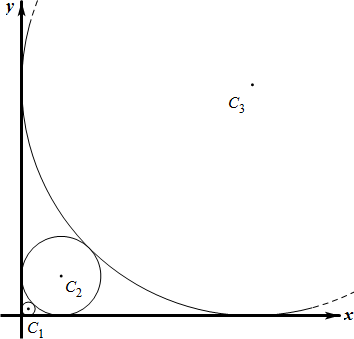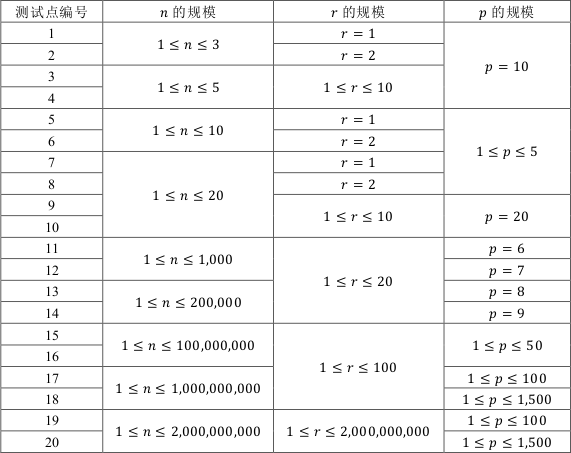# YZOJ P2697 画圆

• ### 题目描述

$$Alkri$$ 想在平面直角坐标系的第一象限中依次画 $$n$$ 个与两坐标轴均相切的圆，其中，第 $$1$$ 个圆的半径为 $$r$$，之后的每个圆都比上一个圆大，且与上一个圆相切，也就是说，对所有整数 $$2 \leq i \leq n$$，第 $$i$$ 个圆的半径大于第 $$i-1$$ 个圆的半径且与第 $$i-1$$个圆相切。• ### 数据规模与约定…

# YZOJ P3314 计算器

• ### 题目描述

< 表达式 > ：  < 运算数 1>< 运算符 1>< 运算数 2>< 运算符 2> $$\cdots$$ < 运算符 $$k-1$$>< 运算数 $$k$$>（ $$k$$ 为正整数）

• ### 数据规模与约定

$$1 \leq \left| s \right| \leq 10^4$$

…